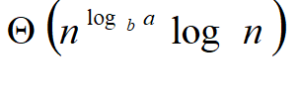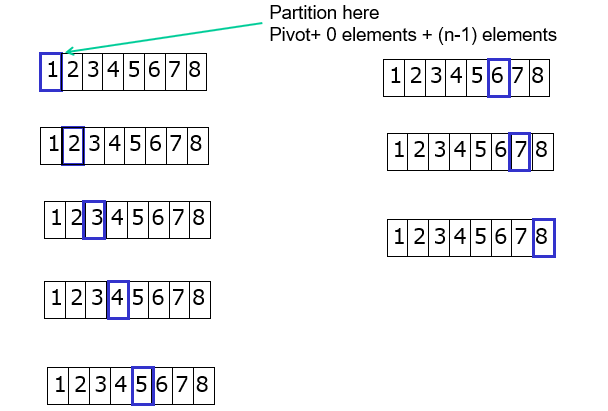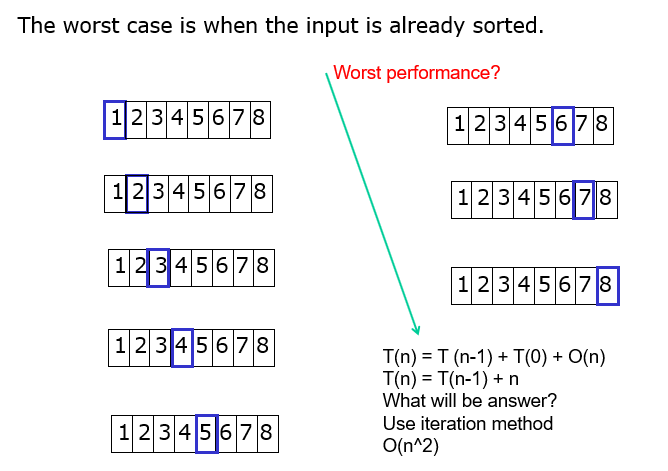# Quick Sort

Quick Sort :  Based on Divide and Conquer paradigm.

• One of the fastest in-memory sorting algorithms (if not the fastest)
• is a very efficient sorting algorithm
• designed by C.A.R.Hoare in 1960.
• Consists of two phases:
• Partition phase
• Sort phase

Steps…

1. Divide: Pick a pivot element and rearrange the array so that

–  all elements to the left of the pivot are

smaller than the pivot.

–  all elements to the right of the pivot are larger

than the pivot.

1. Conquer: Recursively quicksort the left and right subarrays.

3:Combine: since subarrays are sorted in place, no work is needed to combine them, array is now sorted.

## Quicksort – Partition phase

• Goals:
• Select pivot value
• Move everything less than pivot value to the left of it
• Move everything greater than pivot value to the right of it

## Algorithm:  Quick Sort

Procedure  QuickSort ( A, l, r )

if ( r > l ) then

j ¬ partition  ( A, l, r );

QuickSort ( A, l, j – 1 );

QuickSort ( A, j + 1 , r );

end of if.

Function Partition (A, l, r )

v ¬ a[ l ];   i ¬ lj ¬ r;

while i<j

while (A[i]<=v && i<r)  i++;

while (A[j]>v && j>l)  j–;

if (i<j) then swap (a[ i ], a[ j ]);

A [ l ] = a[ j ];  a[ j ] ¬ v;

return (  j );

There are various algorithms for partition.

Above Algorithm does not need an extra array.

Only 3 variables v,i,  and  j are used.

## Time Complexity: Best case/average case

• Recurrence Relation

T(n)=2T(n/2) + n

Using Master Theorem applying case 2:So time complexity is O(nlogn)

• Why is quick sort better than merge sort?
• Better memory consumption

The worst case is when the input is already sorted.## Randomized Quick Sort

• Randomize the input data before giving it to Quick Sort. OR
• In the partition phase, rather than choosing first value to be the pivot value, choose a RANDOM value to be pivot value.
• This makes Quick Sort run time independent of input ordering
• So Worst case won’t happen for SORTED inputs but may only happen by worseness of random number generator.

## C++ code  for quick sort using recursive function

#include <cstdlib>
#include <iostream>
#include<time.h>
#include<conio.h>
#define size 10

using namespace std;

void swap(int&a,int&b)
{
int tmp=a; a=b; b=tmp;
}

void quicksort(int a[],int left,int right)
{
int i=left,j=right,x=(left+right)/2,pivot=a[x];

while(1)
{
while(a[i]<pivot) i++;
if(i>x)break;
while(a[j]>pivot) j–;
if(j<x)break;
if(i<=j)
{
swap(a[i],a[j]);
i++;
j–;
}
}
if(j>left)
quicksort(a,left,j);

if(i<right)
quicksort(a,i,right);
}

int main()
{
srand(time(0));
int a[size],j=10;
for(int i=0;i<size;i++)
a[i]=rand()%100;

cout<<“Original Array: “;
for(int i=0;i<size;i++)
cout<<a[i]<<” “;
cout<<endl<<endl;

quicksort(a,0,size-1);
cout<<“Sorted Array: “;
for(int i=0;i<size;i++)
cout<<a[i]<<” “;
cout<<endl<<endl;
getch();
return EXIT_SUCCESS;
}